Monday 13th July 2020

CBSE Guess > Papers > Question Papers > Class XII > 2004 > Maths > Compartment Outside Delhi Set-II

MATHS (Set II—Compartment Outside Delhi)

Except for the following questions, all the remaining questions have been asked in Set I .

SECTION - A

Q. 1. If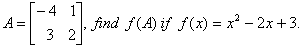Q. 3. Solve the following differentia equation: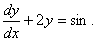Q. 10. Differentiate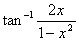with respect to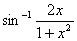Q. 13. Evaluate: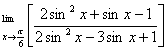Q. 15. If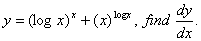f

Or

Differentiate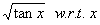from first principles.

Q. 18. Using integration, find the area of the region in the first quadrant enclosed by the x-axis, the line an the circle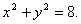SECTION - B

Q. 21. Using vectors, prove that if the diagonals of a parallelogram are equal in length it is a rectangle.

SECTION - C

Q. 19. A box contains 4 gold and 3 silver coins. Another box contains 3 gold and 5 silver coins. A box is chosen at random and a coin is drawn from it. If the selected coin is a gold coin, find the probability that it was drawn from the second box.

 Maths 2004 Question Papers Class XII Delhi Outside Delhi Compartment Delhi Compartment Outside DelhiSet ISet ISet ISet ISet IISet IISet IISet IISet IIISet III

CBSE 2004 Question Papers Class XII